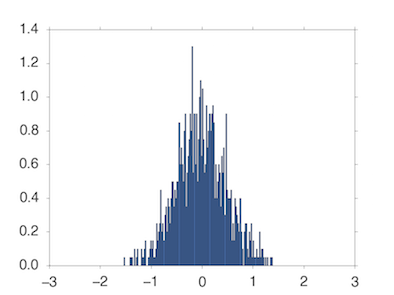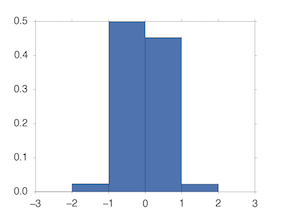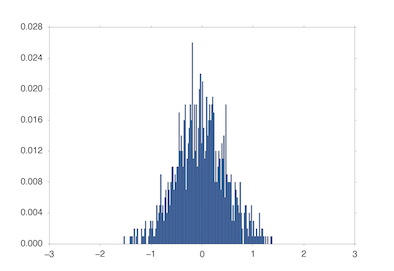# Histogram with matplotlib

A note of what I was addicted to when creating a histogram with matplotlib

# pitfalls of normed

Note that matplotlib.axes.Axes.hist () or matplotlib.pyplot.hist (), which is used to create histograms with matplotlib, has a terrifying pitfall. When creating a histogram, we often add the option normed = 1 to normalize the frequency. However, despite this option, the histogram may look like the one below.

``````import numpy as np
import matplotlib.pyplot as plt
np.random.seed(0)
nor = np.random.normal(0,0.5,1000)
plt.hist(nor,normed=1,range=(-3,3),bins=300)
plt.savefig("test.pdf")
``````Eh, the y-axis scale value is greater than 1. ..

At first, I thought this was a bug and tried to force a histogram using barplot. But it's too much trouble. I thought it was a seaborn distroplot, but it didn't work because I had the same problem. When I thought it was a mystery, I realized that there was one.

``````import numpy as np
import matplotlib.pyplot as plt
np.random.seed(0)
nor = np.random.normal(0,0.5,1000)
plt.hist(nor,normed=1,range=(-3,3),bins=6)
plt.savefig("test.pdf")
``````If the width of __bin is 1, it works normally. __ __ No way __ ,, How, __bin is normalized so that the total area of __bin is 1. Actually, if you look at the details of the data using numpy's histogram function (the behavior is the same as matplotlib's histogram)

``````import numpy as np
np.random.seed(0)
nor = np.random.normal(0,0.5,1000)
hist,pos = np.histogram(nor,normed=1,range=(-3,3),bins=300)
print(np.sum(hist))
#50.0
print(np.sum(hist)*6.0/300)
#1.0
``````

Will be. Therefore, you can rewrite the code as follows.

``````import numpy as np
import matplotlib.pyplot as plt
np.random.seed(0)
nor = np.random.normal(0,0.5,1000)
binnum = 300
fig = plt.figure()
ax = plt.subplot(111)
ax.hist(nor,normed=1,range=(-3,3),bins=binnum)
ax_yticklocs = ax.yaxis.get_ticklocs()#Get scale information
ax_yticklocs = list(map(lambda x: x * len(range(-3,3))*1.0/binnum, ax_yticklocs))#Multiply the value of the original scale by the width of bin
ax.yaxis.set_ticklabels(list(map(lambda x: "%0.2f" % x, ax_yticklocs)))Show fixed scale
plt.savefig("test.pdf")
``````You should probably have the scale you want. However, this implementation is confusing ...